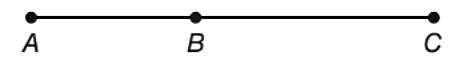Chapter 1.2, Problem 35E### Elementary Geometry for College St...

6th Edition
Daniel C. Alexander + 1 other
ISBN: 9781285195698

#### Solutions

Chapter
Section### Elementary Geometry for College St...

6th Edition
Daniel C. Alexander + 1 other
ISBN: 9781285195698
Textbook Problem
1 views

# In Exercises 35 to 38, with A - B - C on A C ¯ , it follows that A B + B C = A C .Find A C if A B = 9 and B C = 13 .

To determine

To find:

The value of AC

Explanation

Given, AB+BC=AC, AB=9,BC=13

Calculation:

Here, AB=9 and <

### Still sussing out bartleby?

Check out a sample textbook solution.

See a sample solution

#### The Solution to Your Study Problems

Bartleby provides explanations to thousands of textbook problems written by our experts, many with advanced degrees!

Get Started